# Resistors, Capacitors, and Inductors

The average person uses a lot of electricity in the many things they do every day. We are used to being able to turn on a light, watch the television, use our mobile devices, and stream music or videos. Because electricity is so much a part of our lives, we should know something about it.

There are three basic components of electric circuits and each affects the current and can change the behavior of a circuit.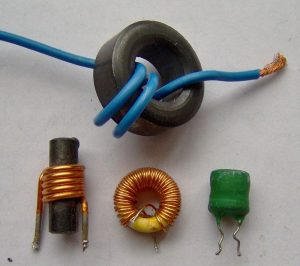The three components ae: resistors, capacitors, and inductors. Each one of these components plays a role in the behavior of an electronic circuit. Each of these three components are represented with specific symbols and alphabetic letters, as well as measurement names.

### Resistors

As you might guess by the name, resistors have a certain amount of resistance or opposition to the electric current. The higher the resistance that a resistor has the less current it allows to flow through.

Resistance is measured in units that care called “ohms” and its abbreviation symbol is the Greek omega Ω.  There are various purposes for resistors in electronic circuits.

In Ohm’s Law, resistance equals the voltage divided by the current. The formula for this is:

R = V/I

R=resistance, V=voltage, I= current

The symbol used to represent resistance is a zigzag line:

Resistor Symbol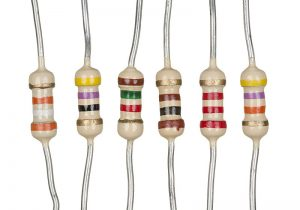### Capacitors

Electric current is the flow of electrons that creates electric charges. In the same way that we can store water, the electric charges can be stored. The component used to store the electrical charges is called the capacitor.

The ability to be able to store the charges is called “capacitance” and is measured in “Farads” (abbreviated as “F”) The higher the capacitance that a capacitor has, the more storage of electric charges it can have.  Another way of thinking of this is to use the word “capacity.”

Capacitance is defined in the formula:

C = q/V
q=the charge in coulombs, V=voltage.

A DC (direct current) circuit has a capacitor that becomes an open circuit that blocks any DC current from passing the capacitor. Only alternating current (AC) will pass through a capacitor.

The symbol for capacitance is two parallel lines; and in some cases one of the lines may be curved. Capacitance is also symbolized with the letter “C.”

Capacitor Symbol

### Inductors

Opposing a change in current is called “inductance.”  When an electrical component has inductance, it’s called an inductor. DC (direct current) doesn’t have any change in the flow of electricity.

Inductors are compared to small short circuits to the DC (direct current), and they provide a kind of opposition similar to resistors in AC (alternating current).

A definition of inductance is that they have the ability to change the flow of current and generate electromotive force. Simple inductors that are used in electronic circuits oppose or reduce the amount of change in the electric current.

When current is constant in DC (direct current) the inductor that looks like a wire doesn’t have any effect. Inductance will only have an effect when the current changes such as in an AC (alternating current) circuit.

Inductance in measured in a “Henrys,” and is represented by the letter “L” and the symbol that looks like a bunch of coils.

Inductor Symbol

## Fun Facts about Resistors, Capacitors, and Inductors

Capacitors are measured in “Farads,” which are named after Michael Faraday, an English physicist.

The Ohm measurement is named after Georg Ohm, a German physicist.

Inductance is measured in “Henrys” which are named after Joseph Henry, an American scientist.

Sophisticated passive filters use a combination of capacitors, inductors, and resistors so that they only allow electronic signals that are in specific frequencies to pass through.

The polarity reverses of AC current don’t allow capacitors to store electrical charges; they are constantly storing and then draining.

### Q&A:

What is the definition of resistors in electronic circuits?
resistors have a certain amount of resistance or opposition to the electric current

How are resistors measured?
Ohms

What is the definition of a capacitor in electronic circuits?
The component used to store electrical charges

How are capacitors measured?
•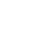History
•Science
•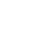Geography
•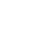Biography
•US History